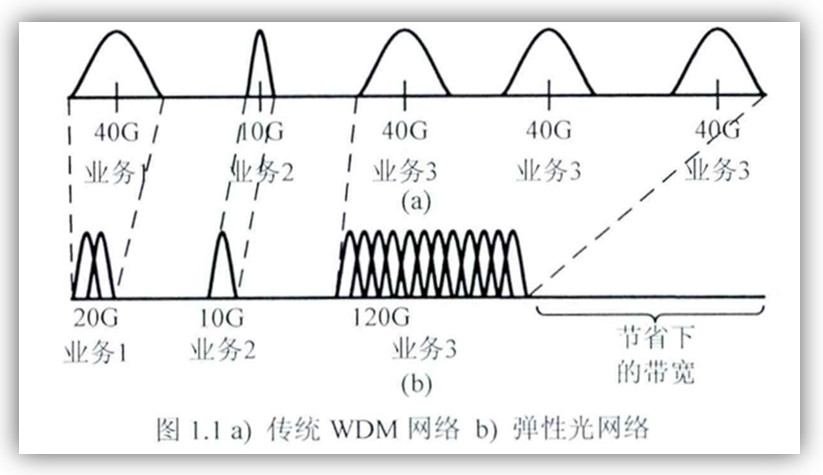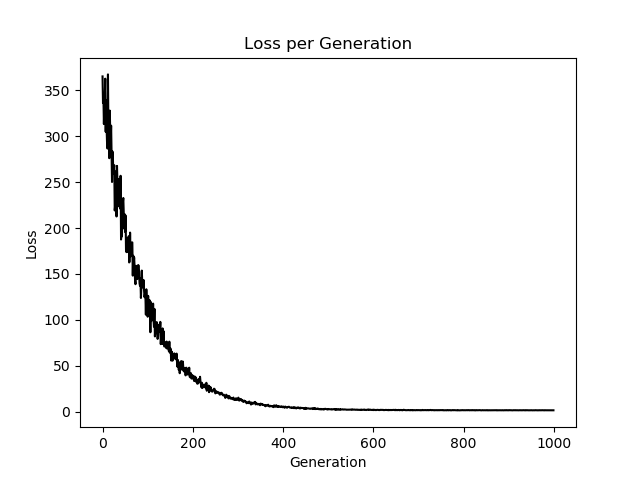• ## 弹性网络

千次阅读 2018-07-26 22:56:31
ElasticNet 是一种使用L1和L2先验作为正则化矩阵的线性回归模型....当多个特征和另一个特征相关的时候弹性网络非常有用。Lasso 倾向于随机选择其中一个，而弹性网络更倾向于选择两个. 在实践中，Lasso...
弹性网络在线性回归的基础上加上了一个

l

2

l_2

和一个

l

1

l_1

。这种组合用于只有很少的权重非零的稀疏模型，比如Lasso, 但是又能保持Ridge 的正则化属性。
当多个特征和另一个特征相关的时候弹性网络非常有用。Lasso 倾向于随机选择其中一个，而弹性网络更倾向于选择两个.
在实践中，Lasso 和 Ridge 之间权衡的一个优势是它允许在循环过程（Under rotate）中继承 Ridge 的稳定性.
损失函数：

J

(

θ

)

=

1

2

m

∣

∣

X

θ

−

y

∣

∣

2

2

+

α

ρ

∣

∣

θ

∣

∣

1

+

α

(

1

−

ρ

)

2

∣

∣

θ

∣

∣

2

2

J(\theta)=\frac 1 {2m}||X\theta-y||^2_2+\alpha \rho||\theta||_1+\frac {\alpha(1- \rho)}2||\theta||_2^2

Python实现 TODO
import numpy as np


展开全文• 主要介绍了用tensorflow实现弹性网络回归算法
• 文章目录弹性网络架构基本元素架构图基本原理结论 弹性网络 架构 基本元素 架构图 基本原理 弹性网络划分了最小频隙单位12.5GHz，相对于传统WDM的固定信道间隔的波长分配，为业务需求带宽分配适当...


文章目录
弹性光网络架构基本元素架构图
基本原理结论

弹性光网络
架构
基本元素
架构图基本原理
弹性光网络划分了最小频隙单位12.5GHz，相对于传统WDM的固定信道间隔的波长分配，为业务需求带宽分配适当数量的彼此相邻的单位频隙，实现了按需分配。比如某业务需要25GHz的带宽，使用传统的WDM只能分配一个50GHz的固定信道间隔的波长信道给业务，造成了不必要的带宽浪费，而弹性光网络只需要分配两个相邻的频隙(25GHz)给业务，节省了带宽资源，提高了频谱使用效率。使用了OFDM调制技术后，当业务到来时，将业务调制到适当数量的彼此邻接的光子载波上，所有光子载波的带宽都相等，都等于12.5GHz，由于相邻光子载波间彼此正交，所以允许彼此相互重叠1/2的带宽(6.25GHz)而不会对对方造成干扰。子载波的重叠性使得同样的业务带宽需求相对于传统的WDM占用更少的频谱资源，却完成了同样的传输效果。引入了最小单位频隙，使得业务分配更加灵活高效，子波长业务(如10GHz，业务分割)，超波长业务(超级信道，如400GHz，业务聚合)的灵活适配成为可能，而传统的WDM只能通过分配一个冗余过多的波长或多个波长间接适配。相对于子波长，传统的WDM就像是我需要一个床铺的空间大小，你却给我一个寝室(波长)的空间来用，我需要多花钱来买不必要的空间，而本来一个寝室的空间能给四个人用，空间提供商能用一个寝室空间为四个人提供服务赚四个人的钱，现在却只能服务我一个人，而空间有限，造成空间浪费。相对于超波长，传统的WDM就像是我需要两个半寝室大小空间的办公室，你却只能给我三个寝室的空间来用，三个寝室间的两个墙壁(相邻波长间的保护带宽)造成了空间浪费，多给的半个寝室空间又造成了空间浪费。带宽的弹性变化(压缩和扩展)是弹性光网络的一大重要特性。比如随着时间的变化，某业务需要扩展，或者缩小，已分配带宽的弹性变化满足了这种灵活的需求。
结论
展开全文•研究论文
• 弹性网络中虚拟网络映射的常用算法，包括频谱分配 首次命中 KSP等算法
• 在每年的淘宝网购狂欢节中，IT系统都是其背后最关键的支撑平台。而网络基础设施则又是IT系统赖以完美运行的幕后英雄。让我们撩开神秘的面纱，一睹淘宝弹性网络架构的真实容颜。
• 网络游戏-弹性网络中动态虚拟网络映射算法研究.zip
• 针对弹性网络中虚拟网络映射的资源消耗问题, 综合考虑节点、链路对虚拟网络映射资源需求的影响, 提出了节点优先的虚拟网络映射算法。所提算法先对虚拟节点依据节点权值进行排序, 然后为虚拟节点分配满足资源需求的...
• 本文使用多线性回归的方法实现弹性网络回归算法，以iris数据集为训练数据，用花瓣长度、花瓣宽度和花萼宽度三个特征预测花萼长度。 # Elastic Net Regression # 弹性网络回归 #-------------------------...
弹性网络回归算法（Elastic Net Regression）是综合lasso回归和岭回归的一种回归算法，通过在损失函数中增加L1和L2正则项。
本文使用多线性回归的方法实现弹性网络回归算法，以iris数据集为训练数据，用花瓣长度、花瓣宽度和花萼宽度三个特征预测花萼长度。
# Elastic Net Regression
# 弹性网络回归
#----------------------------------
#
# This function shows how to use TensorFlow to
# solve elastic net regression.
# y = Ax + b
#
# We will use the iris data, specifically:
#  y = Sepal Length
#  x = Pedal Length, Petal Width, Sepal Width

import matplotlib.pyplot as plt
import numpy as np
import tensorflow as tf
from sklearn import datasets
from tensorflow.python.framework import ops

###
# Set up for TensorFlow
###

ops.reset_default_graph()

# Create graph
sess = tf.Session()

###
# Obtain data
###

# iris.data = [(Sepal Length, Sepal Width, Petal Length, Petal Width)]
x_vals = np.array([[x, x, x] for x in iris.data])
y_vals = np.array([y for y in iris.data])

###
# Setup model
###

# make results reproducible
seed = 13
np.random.seed(seed)
tf.set_random_seed(seed)

# Declare batch size
batch_size = 50

# Initialize placeholders
x_data = tf.placeholder(shape=[None, 3], dtype=tf.float32)
y_target = tf.placeholder(shape=[None, 1], dtype=tf.float32)

# Create variables for linear regression
A = tf.Variable(tf.random_normal(shape=[3,1]))
b = tf.Variable(tf.random_normal(shape=[1,1]))

# Declare model operations

# Declare the elastic net loss function
# 对于弹性网络回归算法，损失函数包含斜率的L1正则和L2正则。
# 创建L1和L2正则项，然后加入到损失函数中
elastic_param1 = tf.constant(1.)
elastic_param2 = tf.constant(1.)
l1_a_loss = tf.reduce_mean(tf.abs(A))
l2_a_loss = tf.reduce_mean(tf.square(A))
e1_term = tf.multiply(elastic_param1, l1_a_loss)
e2_term = tf.multiply(elastic_param2, l2_a_loss)

# Declare optimizer
train_step = my_opt.minimize(loss)

###
# Train model
###

# Initialize variables
init = tf.global_variables_initializer()
sess.run(init)

# Training loop
loss_vec = []
for i in range(1000):
rand_index = np.random.choice(len(x_vals), size=batch_size)
rand_x = x_vals[rand_index]
rand_y = np.transpose([y_vals[rand_index]])
sess.run(train_step, feed_dict={x_data: rand_x, y_target: rand_y})
temp_loss = sess.run(loss, feed_dict={x_data: rand_x, y_target: rand_y})
loss_vec.append(temp_loss)
if (i+1)%250==0:
print('Step #' + str(i+1) + ' A = ' + str(sess.run(A)) + ' b = ' + str(sess.run(b)))
print('Loss = ' + str(temp_loss))

###
# Extract model results
###

# Get the optimal coefficients
[[sw_coef], [pl_coef], [pw_ceof]] = sess.run(A)
[y_intercept] = sess.run(b)

###
# Plot results
###

# Plot loss over time
plt.plot(loss_vec, 'k-')
plt.title('Loss per Generation')
plt.xlabel('Generation')
plt.ylabel('Loss')
plt.show()


结果：
Step #250 A = [[ 0.93870646]
[-0.37139279]
[ 0.27290201]] b = [[-0.36246276]]
Loss = [ 23.83867645]
Step #500 A = [[ 1.26683569]
[ 0.14753909]
[ 0.42754883]] b = [[-0.2424359]]
Loss = [ 2.98364353]
Step #750 A = [[ 1.33217096]
[ 0.28339788]
[ 0.45837855]] b = [[-0.20850581]]
Loss = [ 1.82120061]
Step #1000 A = [[ 1.33412337]
[ 0.32563502]
[ 0.45811999]] b = [[-0.19569117]]
Loss = [ 1.64923525]展开全文• 在传统供应网络和多源供应网络设计的基础上,结合战略应急库存和实物期权两种应急供应方法,建立了基于应急供应的弹性供应网络模型,并进行了算例验证.研究结果表明,与传统的供应网络模型相比,该模型更具有现实意义,...
• 使用弹性网络正则化执行逻辑回归的通用函数。 用法： call_generic_elasticnet_glm_predict() 此函数加载玩具数据并使用弹性网络正则化执行逻辑回归。 改编自： ...matlab
• 网络控制系统在遭遇恶意攻击下的弹性控制，利用云平台
• 网络游戏-弹性网络随机路由路径生成方法.zip
• 为解决弹性网络中虚拟网络功能服务链的选路和虚拟网络功能配置问题,建立了一个以网络中最大占用频隙号、网络中占用频隙数,以及配置的虚拟网络功能数目最小化为目标的全局约束优化模型。将虚拟网络功能划分为具有...
• 弹性网络 模型 弹性网络是一种使用 L1， L2 范数作为先验正则项训练的线性回归模型。 这种组合允许学习到一个只有少量参数是非零稀疏的模型，就像 Lasso 一样，但是它仍然保持一些像 Ridge 的正则性质。我们可利用...
弹性网络
模型
弹性网络是一种使用 L1， L2 范数作为先验正则项训练的线性回归模型。 这种组合允许学习到一个只有少量参数是非零稀疏的模型，就像 Lasso 一样，但是它仍然保持一些像 Ridge 的正则性质。我们可利用 l1_ratio 参数控制 L1 和 L2 的凸组合。
弹性网络在很多特征互相联系的情况下是非常有用的。Lasso 很可能只随机考虑这些特征中的一个，而弹性网络更倾向于选择两个。
在实践中，Lasso 和 Ridge 之间权衡的一个优势是它允许在循环过程（Under rotate）中继承 Ridge 的稳定性。
最小化的目标函数是：

minw12n||Xw−y||22+αρ||w||1+α(1−ρ)2||w||22

min

w

1

2

n

|

|

X

w

−

y

|

|

2

2

+

α

ρ

|

|

w

|

|

1

+

α

(

1

−

ρ

)

2

|

|

w

|

|

2

2

\min_w \frac{1}{2n}||Xw-y||_2^2+\alpha\rho||w||_1+\frac{\alpha(1-\rho)}{2}||w||_2^2

算法：
坐标下降算法（coordinate descent ）
令

L(w)==12n||Xw−y||22+αρ||w||1+α(1−ρ)2||w||2212n∑i(∑jxijwj−yi)2+αρ∑i|wi|+α(1−ρ)2∑iw2i

L

(

w

)

=

1

2

n

|

|

X

w

−

y

|

|

2

2

+

α

ρ

|

|

w

|

|

1

+

α

(

1

−

ρ

)

2

|

|

w

|

|

2

2

=

1

2

n

∑

i

(

∑

j

x

i

j

w

j

−

y

i

)

2

+

α

ρ

∑

i

|

w

i

|

+

α

(

1

−

ρ

)

2

∑

i

w

i

2

\begin{eqnarray*}
L(w)&=& \frac{1}{2n}||Xw-y||_2^2+\alpha\rho||w||_1+\frac{\alpha(1-\rho)}{2}||w||_2^2\\
&=&\frac{1}{2n}\sum_i(\sum_j x_{ij}w_j-y_i)^2+\alpha\rho\sum_i|w_i|+\frac{\alpha(1-\rho)}{2}\sum_iw_i^2
\end{eqnarray*}

其次导数为：

∂L∂wk=====1n∑ixik(∑jxijwj−yi)+α(1−ρ)wk+αρ∂|wk|1n(∑ix2ik)wk+1n∑ixik(∑j≠kxijwj−yi)+α(1−ρ)wk+αρ∂|wk|(1n(∑ix2ik)+α(1−ρ))wk−1n∑ixik(yi−∑j≠kxijwj)+αρ∂|wk|awk−b+αρ∂|wk|⎧⎩⎨awk−b+αρ[−b−αρ,−b+αρ]awk−b−αρwk>0wk=0wk<0

∂

L

∂

w

k

=

1

n

∑

i

x

i

k

(

∑

j

x

i

j

w

j

−

y

i

)

+

α

(

1

−

ρ

)

w

k

+

α

ρ

∂

|

w

k

|

=

1

n

(

∑

i

x

i

k

2

)

w

k

+

1

n

∑

i

x

i

k

(

∑

j

≠

k

x

i

j

w

j

−

y

i

)

+

α

(

1

−

ρ

)

w

k

+

α

ρ

∂

|

w

k

|

=

(

1

n

(

∑

i

x

i

k

2

)

+

α

(

1

−

ρ

)

)

w

k

−

1

n

∑

i

x

i

k

(

y

i

−

∑

j

≠

k

x

i

j

w

j

)

+

α

ρ

∂

|

w

k

|

=

a

w

k

−

b

+

α

ρ

∂

|

w

k

|

=

{

a

w

k

−

b

+

α

ρ

w

k

>

0

[

−

b

−

α

ρ

,

−

b

+

α

ρ

]

w

k

=

0

a

w

k

−

b

−

α

ρ

w

k

<

0

\begin{eqnarray*}
\frac{\partial L}{\partial w_k}&=&\frac{1}{n}\sum_ix_{ik}(\sum_jx_{ij}w_j-y_i)+\alpha(1-\rho)w_k+\alpha\rho\partial|w_k| \\
&=&\frac{1}{n}(\sum_ix_{ik}^2)w_k+\frac{1}{n}\sum_ix_{ik}(\sum_{j\neq k}x_{ij}w_j-y_i)+\alpha(1-\rho)w_k+\alpha\rho\partial|w_k| \\
&=&(\frac{1}{n}(\sum_ix_{ik}^2)+\alpha(1-\rho))w_k-\frac{1}{n}\sum_ix_{ik}(y_i-\sum_{j\neq k}x_{ij}w_j)+\alpha\rho\partial|w_k| \\
&=&aw_k-b+\alpha\rho\partial|w_k| \\
&=&
\begin{cases}
aw_k-b+\alpha\rho & w_k>0\\
[-b-\alpha\rho, -b+\alpha\rho] & w_k = 0 \\
awk-b-\alpha\rho & w_k<0
\end{cases}
\end{eqnarray*}

其中：

a=1n(∑ix2ik)+α(1−ρ)b=1n∑ixik(yi−∑j≠kxijwj)

a

=

1

n

(

∑

i

x

i

k

2

)

+

α

(

1

−

ρ

)

b

=

1

n

∑

i

x

i

k

(

y

i

−

∑

j

≠

k

x

i

j

w

j

)

a=\frac{1}{n}(\sum_ix_{ik}^2)+\alpha(1-\rho)\\
b=\frac{1}{n}\sum_ix_{ik}(y_i-\sum_{j\neq k}x_{ij}w_j)

令次导数为零可得：

wk=⎧⎩⎨⎪⎪⎪⎪b−αρa0b+αρab>αρ−αρ≤b≤αρb<−αρ(*)

(*)

w

k

=

{

b

−

α

ρ

a

b

>

α

ρ

0

−

α

ρ

≤

b

≤

α

ρ

b

+

α

ρ

a

b

<

−

α

ρ

w_k=
\begin{cases}
\frac{b-\alpha\rho}{a} & b>\alpha\rho\\
0 & -\alpha\rho \leq b \leq \alpha\rho \\
\frac{b+\alpha\rho}{a} & b<-\alpha\rho
\end{cases} \tag{*}

算法过程：

选择参数的一个维度

wk

w

k

$w_k$，可以是随机也可以是顺序用(*)式更新

wk

w

k

$w_k$
展开全文• 弹性网络以灵活利用频谱为特征，可以根据用户需要和业务量大小动态分配适量的频谱资源并配置相应的调制格式，有效克服了波分复用光网络的缺陷。同时，弹性网络中的多播路由和频谱分配以及网络的生存性问题也变得...多播 路由
• 星形热弹性网络系统的稳定性分析，韩忠杰，许跟起，本文考察了一类非均匀热弹性网络系统的动力学行为。不同于过去讨论单一热弹性系统,本文从网络观点考虑热弹性动力学问题。假设网�
•研究论文...